Published: 30 September 2017

# A hydraulic fault diagnosis method based on sliding-window spectrum feature and deep belief network

Xinqing Wang1
Jie Huang2
Guoting Ren3
Dong Wang4
1, 2, 3, 4College of Field Engineering, People’s Liberation Army University of Science and Technology, Nanjing, China
Corresponding Author:
Jie Huang
Views 247

#### Abstract

The vibration signal of hydraulic system contains abundant state information, so vibration testing technology is an effective way to realize the fault diagnosis of hydraulic system. However, the mapping relation between signal characteristic and system state is complex and the expression meaning of characteristic is obscure, which brings a great challenge to the hydraulic fault diagnosis. The DBN, a newly proposed deep learning model, has an advantage of autonomously learning and reasoning. And it is good at studying the concealed representation of data and highlighting the feature expression. So, it is contributive to deal with the problems of large capacity data like high dimension, redundancy, and nonlinear etc. Therefore, DBN is chosen as the fault diagnosis method in this paper. Meanwhile, given that the difficulty in feature extraction of hydraulic vibration signal and the important influence of input feature vector to the diagnosing of DBN, a fast and effectively feature extraction method based on sliding-window spectrum feature (SWSF) is proposed. It is effective in remaining the integrity of feature, avoiding the risking of relative shifting of characteristic spectrum, and decreasing the dimensions of feature vector. The experimental results demonstrate that the combination of SWSF and DBN is a fast and effective approach to realize the fault diagnosis of hydraulic system.

## 1. Introduction

Hydraulic system plays an important role in modern industry equipment, so it is of great significance to accurately detect and diagnose fault of hydraulic equipment in order to ensure its safety running. The structure of hydraulic system and its sealed working characteristic make the hydraulic fault present the following features [1, 2], such as invisibility, susceptible to random factors, the complex mapping relation between signal characteristic and system state, etc. Therefore, it is difficult to judge the fault state through the signal directly, so selecting an appropriate diagnosis method is critical. Deep belief network (DBN), a newly proposed deep neural network by Hinton , has a strong ability of autonomously learning and reasoning. It is able to focus on studying the hiding representation of data and highlighting the expression of characteristic data, so it is contributive to deal with the problems of large capacity data like high dimension, redundancy, and nonlinear etc. DBN can obtain higher level characteristics from the low-level features by means of unsupervised and greedy training, and is widely used in fields of machine learning and pattern recognition . In recent years, the applying of DBN to fault diagnosis problems has drawn more and more attention of scholars. For instance, Tamilselvan  uses DBN for fault diagnosis of aircraft engine based on the health state classification. Shao  employs an optimization DBN in rolling bearing fault diagnosis through several time-domain features. But Tamilselvan adopts the raw data directly, which needs a high quality data. And Shao selects several kinds of features factitiously, which excessively depends on experimenter’s experience.

The essence of fault intelligent recognition is the recognizing of specific characteristics describing the corresponding fault by machine learning method, so feature extraction is critical to realize fault intelligent recognition. When DBN is applied to fault diagnosis, the input feature vectors can be varied, whose availability and dimension have a great influence on the diagnosis accuracy and efficiency of DBN . With the development in vibration testing field, most of the test equipment has the trait of large capacity, high frequency and speediness. Consequently, the original measured signal is usually in great amount and high-dimensional, if the original measured signal is directly taken as the input sample of DBN, it will lead to a complicated processing and a long running time, which is adverse to the real-time and rapid diagnosis. So, a certain preprocessing for the original data is essential to improve the diagnosis accuracy and efficiency of DBN.

Generally speaking, the means of obtaining fault feature can be divided into two kinds. One is directly extracting the parameters of original data in time domain, frequency domain or energy domain [8, 9], such as average, kurtosis, etc. And these parameters are used as input features of DBN. Another is applying the approach of decomposition , such as wavelet decomposition [2, 11], empirical mode decomposition [12, 13], singular value decomposition , etc. Then the further disposing results of decomposed components are taken as input features. As for the first means, it mainly depends on experience, which is susceptible to artificial subjective factors. For another decomposition means, an appropriate decomposition method should be chosen firstly, and then a series of onerous processes are carried on, which have not made full use of the powerful function of such a deep learning model like DBN. Therefore, aiming at the fast and effectively generating of input feature vector, a feature extraction method based on sliding-window spectrum feature (SWSF) is put forward in this paper. This method starts with the spectrum function of original signal, gets the input feature vector of DBN through the approach of sliding window, thereby avoiding the manual process of feature extraction and selection, enhancing the intelligence of recognition process, and giving full play to the strong ability of autonomous learning and reasoning of DBN. Through experimental verification, it indicates that the combination of SWSF method and DBN is a quick and effective approach to realize the fault diagnosis of hydraulic system.

## 2. The DBN principle

### 2.1. The DBN structure

A DBN is a feedforward neural network stacked with several Restricted Boltzmann Machines (RBMs), which consists of a visible layer, a number of hidden layers, and an output layer . As shown in Fig. 1, a DBN with four layers is taken as the example to introduce the principle of DBN. This DBN includes two unsupervised RBMs and one supervised BP classifier. In the DBN, the visible layer used to accept input data and the 1st hidden layer compose the RBM1, the 1st and 2nd hidden layers compose the RBM2, and the 2nd hidden layer and the output layer compose the BP classifier.

Fig. 1Structure diagram of a DBN with two hidden layers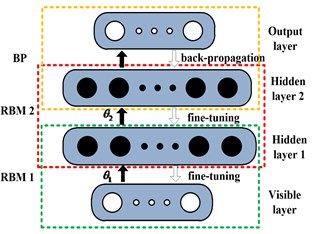The DBN training process consists of two parts, including unsupervised training and supervised training. In the unsupervised training part, the entire network parameters are initialized firstly, and then the RBMs are trained through greedy algorithm. After the training of last RBM is completed, the output of its hidden layer is taken as the input of visible layer of next RBM, and by thus layer-by-layer training, the parameters of each RBM are acquired. In the supervised training part, the label layer of data is brought into training process. The training error is distributed to each RBM by BP classifier through back-propagation algorithm, thereby realizing the updating of the parameters.

### 2.2. The training process of RBM

RBM is a special kind of Markov random field, as well as an energy-based stochastic neural network , its energy function of visible layer $v$ and hidden layer $h$ can be defined as:

1
$E\left(v,h\right)=-{\sum }_{i}{a}_{i}{v}_{i}-{\sum }_{j}{b}_{j}{h}_{j}-{\sum }_{i,j}{v}_{i}{h}_{j}{w}_{ij},$

where ${v}_{i}$ and ${h}_{j}$ are the binary state of visible unit $i$ and hidden unit $j$, ${w}_{ij}$ is the weight between them, ${a}_{i}$ and ${b}_{j}$ are the biases of corresponding units.

Each RBM has one visible layer and one hidden layer, for the two layers, all the visible units and hidden units are connected to each other symmetrically and bidirectionally, and there is no connection between any two units within the same layer. Any of the unit state can be either 1 or 0. The joint probability distribution of visible layer$v$ and hidden layer$h$, and the conditional probabilities of visible unit${v}_{i}$ and hidden unit ${h}_{j}$ can be given as:

2
$P\left(v,h\right)\propto \mathrm{exp}\left({h}^{T}Wv+{b}^{T}v+{a}^{T}h\right),$
3
$P\left({v}_{i}=1|h\right)=\frac{1}{1+\mathrm{e}\mathrm{x}\mathrm{p}\left(-{a}_{i}-{\sum }_{j}^{}{h}_{j}{w}_{ij}\right)},$
4
$P\left({h}_{j}=1|v\right)=\frac{1}{1+\mathrm{e}\mathrm{x}\mathrm{p}\left({-b}_{j}-{\sum }_{i}^{}{v}_{i}{w}_{ij}\right)},$

where $W$ is the weight matrix between visible unit and hidden unit, respectively $a$ and $b$ are the biases matrix of corresponding units.

Researches from experts like Hinton have proved that the parameters $\theta =\left\{W,a,b\right\}$ making $p\left(v,h\right)$ maximized can be obtained by Contrastive Divergence (CD) algorithm. According to CD algorithm, the update rule of the RBM parameters $\theta =\left\{W,a,b\right\}$ is:

5
$∆W={\epsilon }_{W}{\left(〈v{h}^{T}〉}_{data}-{〈v{h}^{T}〉}_{recon}\right),$
6
$∆a={\epsilon }_{a}\left({〈v〉}_{data}-{〈v〉}_{recon}\right),$
7
$∆b={\epsilon }_{b}\left({〈h〉}_{data}-{〈h〉}_{recon}\right),$
8
$W←W+m∆W,$
9
$a←a+m∆a,$
10
$b←b+m∆b,$

where ${\epsilon }_{W}$, ${\epsilon }_{a}$, ${\epsilon }_{b}$ are learning rate of weight, biases of hidden layer and visible layer. ${〈\bullet 〉}_{data}$ refers to the expectation decided by the probability distribution of training data, and is easy to calculate its unbiased estimation. ${〈\bullet 〉}_{recon}$ refers to the expectation decided by the probability distribution of reconstructed data. $m$ is the inertiafactor, which associates the anteroposterior update parameters, and is used to keep the stability of parameter updating.

### 2.3. The advantages of DBN

A large number of experiments prove that, compared with the traditional BP neural network, DBN doesn’t need many labeled training samples, and is no longer limited to the effect of random initialization. RBM is trained layer-by-layer through unsupervised and greedy training, and the training result is taken as the initial value of probability model of supervised learning. This approach can speed up the convergence speed of network, solve the problem of tending to fall into local optimal of BP neural network, improve the learning ability, and provide a technical support for the efficient and deep learning.

## 3. The principle of SWSF method

### 3.1. Problems of applying characteristic spectrum

The spectrum curve of vibration signal contains the system’s state information, when the state varies, the spectrum would also change. Spectrum curve is actually a multimodal function, which usually consists of multiple local peaks . As shown in Fig. 2, the spectrum curve of a hydraulic signal taken from the article’s experiment set, contains nearly 10 significant local peaks. Actually, each local peak is corresponding to a vibration mode. Therefore, in view of the signal’s generation mechanism, a local peak should be a complete feature. In one local peak, pick out the spectrum of maximum amplitude, and define its frequency as the characteristic spectrum of this local peak, such as the frequency of 95.21 Hz in Fig. 3. Obviously, a characteristic spectrum is a vibration mode, and a local peak can be considered as a frequency band decided by the certain characteristic spectrum, and the frequency bands vary in width.

In the fault diagnosis based on vibration testing technology, the spectrum is often taken as the input features of classifier. In reference , the whole Hilbert envelope spectrum of bearing fault signals are used as the feature vectors of DBN, which may cause the following defects. For example, the frequency band containing characteristic spectrum would be dismembered, which destroys the completeness of feature. And make the dimension of input vector very high, which would add the burden of classifier and increase the running time. Besides, for the same type of test sample, there is the risk of relative shifting of characteristic spectrum. For example, Fig. 3 shows the spectrogram of two samples of the same type, judging from the significant frequencies of the two figures, the frequencies of 383.3 Hz and 385.7 Hz should be the representation of the same fault feature. However, the relative shifting of characteristic spectrum appears as a result of test environment or man-made factors, which would have a negative impact on the subsequent classification process.

Fig. 2The spectrum curve of a hydraulic signal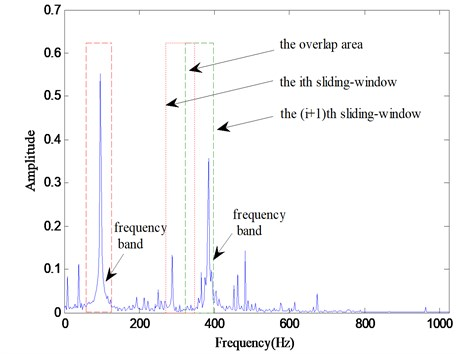Fig. 3The relative shifting of characteristic spectrum in different samples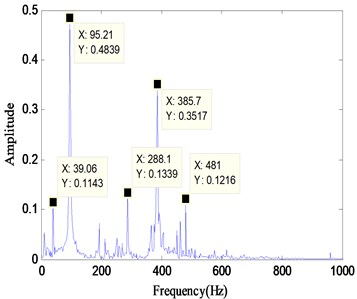a)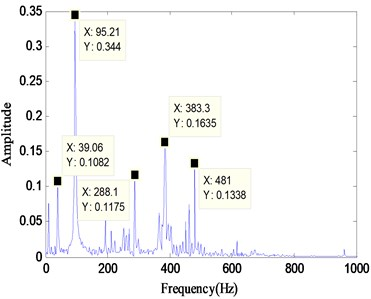b)

Obviously, the division of spectrum curve should be an effective method for above defects. Krakovsky  uses the moving-window discrete Fourier transform as a multichannel filter. Ye  do the modal decomposition by segmenting the spectrum curve. And Ma  uses sliding window FFT algorithm to analysis low frequency oscillation. These papers mainly utilize sliding window for modal analysis or frequency filter, and they split the spectrum function directly into several fragment. But in this paper, the sliding method is used as a feature extraction approach and takes the overlap rate into account. Ideally, by dividing each frequency band corresponding to the characteristic spectrum should be well separated, and these frequency bands would be used as feature vectors. In this case, the integrity of characteristic frequency band is kept, the relative shifting of characteristic spectrum avoided and the dimensions of feature vectors reduced. If adopting the method of equidistant division, it would be difficult for the complete characteristic frequency band to avoid being broken down. If using the method of artificial division, the workload would be increased greatly.

### 3.2. Specific steps of SWSF method

Therefore, in this article, the way of sliding for division is adopted and a feature extraction method based on sliding-window spectrum feature (SWSF) is proposed. The specific steps are as follows.

Step 1, perform spectrum transform for vibration signal $x\left(t\right)$. In this article, calculate the Hilbert envelope spectrum of $x\left(t\right)\text{,}$ and take its unilateral spectrum sequence $F\left(\omega \right)\text{.}$ The subsequent dealing objects are pointing at $F\left(\omega \right)$.

Step 2, determine the width $W$ of sliding-window and the overlap rate $O$ of adjacent sliding-windows, and ensure that the product of $W$ and $O$ is an integer (see Fig. 2).

Step 3, the abscissae of starting and ending of the first sliding-window can be given as:

11
$start\left(1\right)=1,$
12
$end\left(1\right)=W.$

Sum up spectrum sequence of $F\left(\omega \right)$ in the first sliding-window, and get the first sliding spectrum feature $H\left(1\right)$:

13
$H\left(1\right)=\sum _{w=1}^{W}F\left(\omega \right).$

Step 4, conduct the $i$th sliding. Judge whether the ending abscissa of the last sliding is not greater than the sequence length of $F\left(\omega \right)$. If not, the loop terminates. Otherwise, continue sliding. At the moment, the abscissae of starting and ending of the $i$th sliding-window can be given as:

14
$start\left(i\right)=start\left(i-1\right)+\left(1-O\right)W,$
15
$end\left(i\right)=end\left(i-1\right)+\left(1-O\right)W.$

Sum up spectrum sequence of $F\left(\omega \right)$ in the ith sliding-window, and get the $i$th sliding spectrum feature $H\left(i\right)$:

16
$H\left(i\right)=\sum _{w=start\left(i\right)}^{end\left(i\right)}F\left(\omega \right).$

Step 5, obtain the new feature sequence $H\left(v\right)$ of sliding spectrum.

By the feature extraction process SWSF for vibration signal, the feature sequence $H\left(v\right)$ is obtained, which is similar to the histogram sequence in statistical method (hereinafter referred to as sliding spectrum).

The Hilbert envelope spectrum is adopted in the SWSF method, its process can be given as:

1) Perform the Hilbert transform for signal $x\left(t\right)$:

17
$H\left[x\left(t\right)\right]=\frac{1}{\pi }\underset{-\mathrm{\infty }}{\overset{+\mathrm{\infty }}{\int }}\frac{x\left(t\right)}{t-\tau }d\tau .$

2) Calculate the envelope signal $e\left(t\right)$:

18
$e\left(t\right)=\sqrt{{x}^{2}\left(t\right)+{H}^{2}\left[x\left(t\right)\right]}.$

3) Carry on Fourier transform for the envelope signal $x\left(t\right)$, select its unilateral spectrum sequence $E\left(f\right)$ as the envelope spectrum of signal $e\left(t\right)$:

19
$E\left(f\right)=\frac{1}{\pi }\underset{-\mathrm{\infty }}{\overset{+\mathrm{\infty }}{\int }}e\left(t\right)dt.$

### 3.3. An example of applying SWSF

The feature extraction process SWSF is performed for a hydraulic signal under the sliding width of 2 and 4, and overlap rate of 0 respectively. According to the result shown in Fig. 4, the dimensions of sliding spectrum reduce significantly, which are 512 and 256, compared with the 1024 dimensions of the signal’s Hilbert envelope spectrum.

## 4. Experiment validation of the proposed diagnosis solution for hydraulic system

### 4.1. Experiment introduction

The vibration signals used in this paper are measured on the synthetical hydraulic fault experimental platform as shown in Fig. 5, which is entirely made of steel. And these signals are respectively tested under the five states of normal, blockage, leakage, cavitation and impulsion. The measuring points are distributed on the hydraulic cylinder, the sampling frequency is 5000 Hz and the number of sampling points is 2048. Fig. 6 illustrates the time-domain graphs of signals in normal and other four failure states. The figure indicates that the tested vibration signals present different variation rule with different working states, but it is not enough to distinguish these states just depending on the time domain analysis.

Fig. 4Sliding spectrums in two cases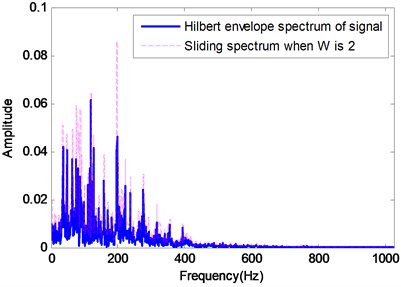a) When $W$ is 2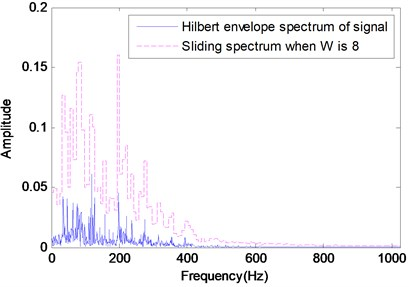b) When $W$ is 8

Fig. 5The synthetical hydraulic fault experimental platform and its schematic diagram. 1 – fuel tank, 2 – suction filter, 3 – control valve of oil-absorbing blockage, 4 – control valve of cavitation, 5 – hydraulic pump, 6 – electromotor, 7 – piezometer, 8 – relief valve, 9 – hand-directional valve, 10 – control valve of leakage, 11 – one-way throttle valve, 12 – flowmeter, 13 – control valve of oil inlet blockage, 14 – control valve of oil outlet blockage, 15 – hydraulic cylinder, 16 – clamping sleeve, 17 – load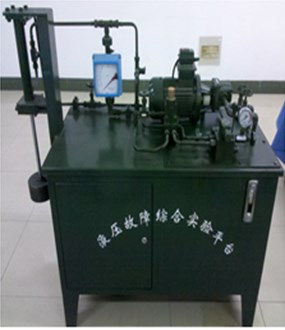a)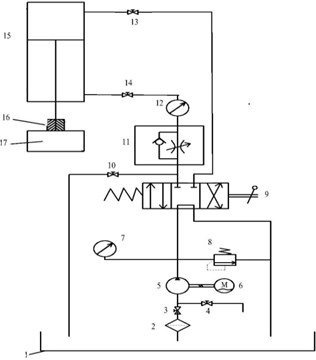b)

### 4.2. Analysis of experiment results

To validate the proposed hydraulic fault diagnosis method combining SWSF and DBN, the data tested from the synthetical hydraulic fault experimental platform is used as the experiment sample set. The training set is consist of 300 samples in normal state, 300 in blockage, 300 in leakage, 300 in cavitation and 180 in impulsion, totaling 1380 samples. And the corresponding testing set is made up of 200 samples in normal state, 200 in blockage, 200 in leakage, 200 in cavitation and 100 in impulsion, totaling 900 samples. Before diagnosis of the experiment sample set, the main structure parameters of DBN need to be determined, and then situations in different sliding width and overlap rate. During the discussing process, the evaluation criteria mostly depend on testing accuracy, while allowing for the elapsed time flexibly.

Fig. 6Time-domain plots of hydraulic vibration signals in five different states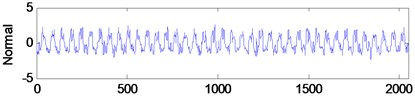a)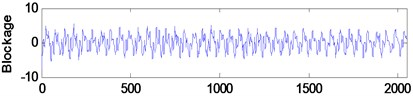b)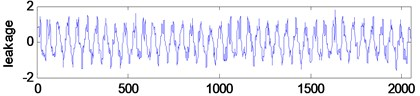c)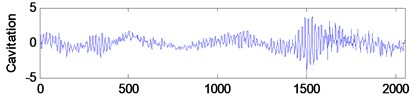d)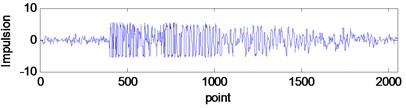e)

### 4.2.1. Discussion on the main structure parameters of DBN

The structure of adopted DBN is $M$-$n$-$n$-5, where $M$ is the dimension of input data, $n$ is the number of units in the hidden layer which usually vary from 10 to 100, and the 5 represents 5 types of states. Obviously, $n$ is an important parameter to for DBN, besides, training times of DBN has a great effect on the classification accuracy and consuming time. Thus, the n and training times are discussed in the following three cases, when the $W$ and $O$ are 2 and 0, 4 and 0.5, 16 and 0.25 respectively.

1) Discussion on the number of hidden units.

When discussing about the value of the number n of hidden units, define the training times as 50 firstly. Then repeating classification testing 10 times, get the averages of these testing results shown in Table 1. By comparison，it is found that the classification accuracy is more better when the number of hidden units is 100, therefore, 100 is decided as the value of n finally.

Table 1Classification results of the DBN with different hidden units

 Classificationaccuracy (%) Number of hidden units 20 40 60 80 100 20 $W/O$ 2/0 93.11 94.56 97.56 97.78 98.00 4/0.5 92.56 96.89 96.00 97.33 97.33 16/0. 25 89.56 89.78 90.00 90.22 91.56

2) Discussion on training times.

According to the previous result, when discussing the training times, choose 100 hidden units and repeat classification testing 10 times. The averages of testing results are displayed in Fig. 7. Combined with the three cases, the curves of classification accuracy tend to be smooth after training times reaches 40. Because the consuming time of DBN’s diagnosis is positive correlated with the training times, so 40 is confirmed as the final value of training times.

Based on the above discussion results, finally, the network structure of DBN can be given as M-100-100-5, and the training times is 40.

Fig. 7Classification results with different training times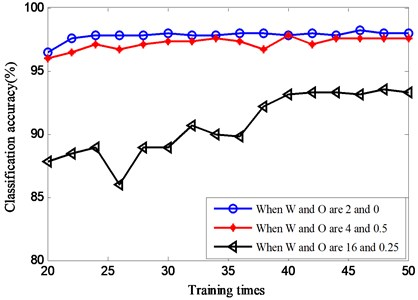### 4.2.2. Cases in different sliding width and overlap rate

The original vibration signals measured in the synthetical hydraulic fault experimental platform are time-domain signals, their length is 2048. By the process of SWSF, the sliding spectrum is obtained, in the next, the sliding spectrum is taken as input sample of the DBN to perform training and classifying, and then what state each sample in is recognized, thereby the fault diagnosis of hydraulic system is realized. It is known that if different sliding width $W$ and overlap rate $O$ are chosen, different sliding spectrum will be got, and the difference mainly reflects in the constitution and dimension of feature, which has a great negative influence on classification accuracy and running time (During the whole running time of DBN, the time spent on the training process occupies a major portion, compared with which, the part time for classifying process is negligible). Then，if the most optimal solution of $W$ and $O$ can be sought out, which can ensure both a high classification accuracy and a less consuming time, thereby achieving the purpose of rapid and real-time diagnosis for hydraulic system. In this article, the choice of $W$ is varied in the range of {1, 2, 4, 8, 16, 32, 64}, and the choice of $O$ is {0, 0.125, 0.25, 0.5}. The detail diagnostic result is shown in Table 2 and Table 3.

Table 2 presents the varied classification result of DBN with different sliding width and overlap rate. There are some vacancies in the table because of the corresponding products of sliding width and overlap rate are not integers, which lead to the sliding process unable to be conducted. It can be seen from Table 2 that the classification accuracy can reach more than 90 % when the sliding width is not more than 16, and the accuracy is above 97 % when the sliding width is 2 or 4 From the horizontal analysis of Table 2, it is found that when the sliding width is greater than 4, the classification accuracy declines gradually with the increase of sliding width. On the contrary, when the sliding width is less than 4, the classification accuracy ascends gradually with the decrease of sliding width.

Table 2Classification results of the DBN with different sliding width and overlap rate

 Classification accuracy (%) $W$ 1 2 4 8 16 32 64 $O$ 0 96.89 98.00 98.44 93.11 89.56 76.89 56.66 0.125 – – – 93.33 91.78 80.67 57.55 0.25 – – 97.11 95.11 92.22 84.67 60.65 0.5 – 97.11 98.00 94.00 90.00 80.00 67.11

Thus, when the sliding width is 4, the difference of features is most obvious and the classification accuracy reaches the highest. The reasons of above result can be described in the following two aspects. On the one hand, when sliding width is small, the relative shifting of characteristic spectrum and some non- characteristic spectrum have an interference in classifying. On the other hand, when sliding width is relatively large, too much spectrum is mixed together, which confuses the distinction between different characteristic spectrum and increases the difficulty of classification. From the vertical analysis of Table 2, it is also found that the overlap rate has little influence on the classification accuracy while the sliding width is small. But once that the sliding width is larger than 4, the classification accuracy when the overlap rate is not 0 are higher than that is 0, and the larger the sliding width is, the more obvious the situation is. It is because the overlap rate is used to dispose the border problem. Each characteristic spectrum does not exist in isolation, this characteristic spectrum and part of its adjacent spectrums belong to one characteristic frequency band decided by one vibration mode. So, in some degree increasing the overlap rate can make the sliding process smoother, which contributes to retaining the integrity of characteristic frequency band and avoiding it being dismembered, thereby improving the classification accuracy to some extent.

Table 3Dimension of sliding spectrum and diagnostic consuming time of DBN when sliding width and overlap rate are different

 Time(s) / dimension $W$ 1 2 4 8 16 32 64 $O$ 0 27.88/1024 18.54/512 13.63/256 11.21/128 9.27/64 8.45/32 8.11/16 0.125 – – – 11.39/146 9.53/170 8.73/36 8.39/18 0.25 – – 15.13/341 12.07/170 10.09/85 9.02/42 8.77/21 0.5 – 27.33/1023 18.13/511 13.83/255 11.12/127 9.45/63 8.74/31

Table 3 describes the changes of the dimension of sliding spectrum and the diagnostic consuming time of DBN. According to Table 3, when the overlap rate remains unchanged, the larger the sliding width is, the less the dimension of sliding spectrum is and the shorter the consuming time is. When the sliding width remains unchanged, the higher the overlap rate is, the higher the dimension of sliding spectrum is and the longer the consuming time is. In a word, with a larger sliding width and a lower overlap rate, the dimension of sliding spectrum will be less and the consuming time shorter. Combining Table 2 and 3, it can be discovered that the difference between the longest consuming time and the shortest one is nearly three times. And when the sliding width is 4, the classification accuracies are about 98 %, and the computation time are 13.63 s,15.13 s and 18.13 s respectively, with a difference of 4.5 s between the minimum and the maximum. So, choose a larger sliding width and a smaller overlap rate in order to decrease the consuming time when the required classification accuracy is guaranteed.

In conclusion, the proposed method combining SWSF and DBN is fast and effective for the intelligent fault diagnosis of this hydraulic experimental sample set. Meanwhile it is very crucial to choose an appropriate sliding width and overlap rate for the certain sample set with taking the classification accuracy and time cost into consideration. And the optimal solution for this hydraulic experimental sample set is that sliding width and overlap rate are 4 and 0 respectively.

### 4.2.3. Change process of features in DBN

In order to study the change process of the feature in each layer of DBN, the input data and output data of two hidden layers are disposed by the principal component analysis (PCA) method. For an alternative visualization, the 3D plots of the first three principal components are shown in Fig. 8, when sliding width is 3 and overlap rata is 0. Fig. 8(a) indicates a poor aggregation with the mixing of a great part features of the 5 states. After the first hidden layer, as shown in Fig. 8(b), there are significant differences between features in each state, except for that between leakage and impulsion, besides a slight mixing is exist between normal and blockage. After the second hidden layer (Fig. 8(c)), in addition to the mixing of a few features between leakage and impulsion, the aggregation extent and the difference between each state improve greatly. The above result suggests that through the feature extracting in hidden layers of DBN layer by layer, the distribution of feature is greatly optimized, the expression ability improved and the discrimination enhanced, thereby performing the following state classifying effectively.

Fig. 8The first three principal components of testing samples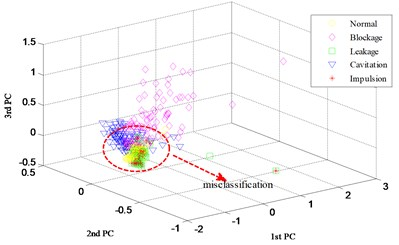a) Input data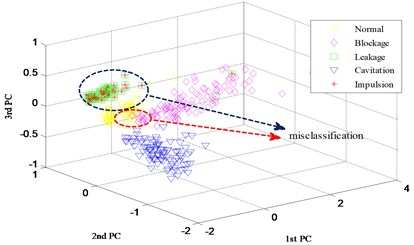b) Output of the 1st hidden layer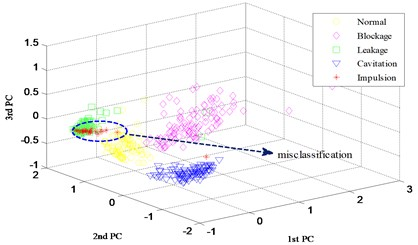c) Output of the 2nd hidden layer

### 4.2.4. Contrastive analysis of different classifiers

Numerous studies have demonstrated that the SVM  is outstanding in shallow network model among kinds of classification algorithms, such as AdaBoost , BP neural network, etc. Therefore, to examine the classification performance of DBN, a representative of deep network model, the contrastive analysis is conducted among DBN, SVM and SVM optimized by PSO. Take the sliding spectrum when sliding width is 3 and overlapping rate is 0 as input features (the data dimension is 256), and get the test results as shown in Table 4. When the classifier is SVM, the parameters using default values, a classification accuracy of 80.67 % is acquired and the consuming time is 1.22 s. When the parameters $c$ and $g$ of SVM are optimized by the PSO algorithm, the classification accuracy raise to 94.67 %, but the occupation time is as long as 830 s. According to the comparison of Table 4, when adopting DBN as classifier, the classification accuracy is obviously the highest and the occupation time is relatively short. It is further evidence that, when feature dimension is high and the mapping relation between feature and state is complex, DBN has an advantage over shallow network models liking SVM.

Table 4Comparison of different classifiers

 Classifier Classification accuracy (%) Consuming time (s) DBN 98.44 13.63 SVM 80.67 1.22 SVM＋PSO 94.67 830

## 5. Conclusions

Aiming at the intractable problem of fault diagnosis for hydraulic system, a novel diagnosis solution combining a new feature extraction method based on sliding-window spectrum feature and DBN is presented. Through experimental verification, it is demonstrated that the proposed diagnosis solution is able to realize the intelligent fault diagnosis of hydraulic system quickly and efficiently.

1) DBN is a deep learning model adopting the greedy learning algorithm layer by layer, which has a powerful learning ability. In the aspects of processing high-dimensional, nonlinear and large volume data, DBN outperform shallow network models such as SVM, which can better represent the complex mapping relation between measured signal and equipment, and is useful to realize the fault diagnosis of hydraulic system.

2) The proposed feature extraction method based on sliding-window spectrum feature is contributive to the rapidly and efficiently generating of input feature vectors of DBN. To some extent, by means of this method, the dismembering of frequency band containing characteristic spectrum can be avoided, the integrity of feature kept, the risking of relative shifting of characteristic spectrum reduced, and the dimensions of feature vectors decreased.

3) For different diagnostic objects, when the SWSF method is adopted, it is needed to choose a suitable sliding solution accordingly. Taking into account both diagnosis accuracy and time cost, as far as possible a larger sliding width and a smaller overlap rate should be selected for a better solution.

#### Cited by

Quantitative Fault Diagnostics of Hydraulic Cylinder Using Particle Filter
Chatter Monitoring of Machining Center Using Head Stock Structural Vibration Analyzed with a 1D Convolutional Neural Network
Deep learning models for predictive maintenance: a survey, comparison, challenges and prospects
Applications of machine learning to machine fault diagnosis: A review and roadmap
An intelligent fault diagnosis approach based on Dempster-Shafer theory for hydraulic valves
An unsupervised and enhanced deep belief network for bearing performance degradation assessment
Deep learning and its applications to machine health monitoring
Convolutional Neural Networks for Electrical Endurance Prediction of Alternating Current Contactors
Limited Data Rolling Bearing Fault Diagnosis With Few-Shot Learning

27 April 2017
Accepted
25 August 2017
Published
30 September 2017
SUBJECTS
Fault diagnosis based on vibration signal analysis
Keywords
hydraulic system
fault diagnosis
feature extraction
sliding-window spectrum feature
deep belief network
Acknowledgements

This research is supported by the National Natural Science Foundation of China (No. 61671470) and the National Key Research and Development Program of China (No. 2016YFC0802900).